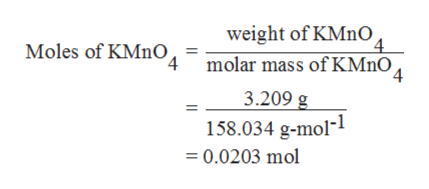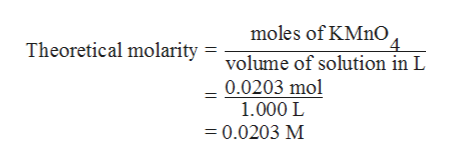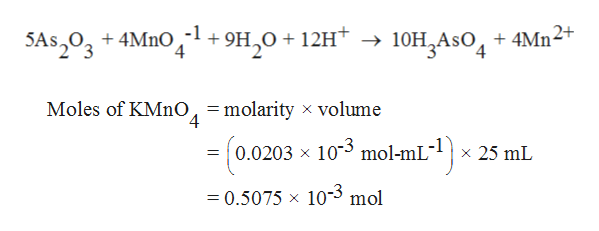# Arsenic(III) oxide (As2O3) is available in pure form and is a useful (but carcinogenic) primary standard for oxidizing agents such as MnO4-. The As2O3 is dissolved in base and then titrated with MnO4- in acidic solution. A small amount of iodide (I - ) or iodate (IO3-) is used to catalyze the reaction between H3AsO3 and MnO4-.As2O3 + 4 OH -  2 HAsO32- + H2OHAsO32- + 2 H+  H3AsO35 H3AsO3 + 2 MnO4- + 6 H+  5 H3AsO4 + 2 Mn2+ + 3 H2O(a) A 3.209 g aliquot of KMnO4 (FM 158.034) was dissolved in 1.000 L of water, heated to cause any reactions with impurities to occur, cooled and filtered. What is the theoretical molarity of this solution if no MnO4- was consumed by impurities?  M(b) What mass of As2O3 (FM 197.84) would be just sufficient to react with 25.00 mL of the KMnO4 solution in part (a)?  g(c) It was found that 0.146 8 g of As2O3 required 29.98 mL of KMnO4 solution for the faint color of unreacted MnO4- to appear. In a blank titration, 0.03 mL of MnO4- was required to produce enough color to be seen. What is the molarity of the permanganate solution?  M

Question
149 views

Arsenic(III) oxide (As2O3) is available in pure form and is a useful (but carcinogenic) primary standard for oxidizing agents such as MnO4-. The As2O3 is dissolved in base and then titrated with MnO4- in acidic solution. A small amount of iodide (I - ) or iodate (IO3-) is used to catalyze the reaction between H3AsO3 and MnO4-.

As2O3 + 4 OH -  2 HAsO32- + H2O
HAsO32- + 2 H+  H3AsO3
5 H3AsO3 + 2 MnO4- + 6 H+  5 H3AsO4 + 2 Mn2+ + 3 H2O
(a) A 3.209 g aliquot of KMnO4 (FM 158.034) was dissolved in 1.000 L of water, heated to cause any reactions with impurities to occur, cooled and filtered. What is the theoretical molarity of this solution if no MnO4- was consumed by impurities?
M

(b) What mass of As2O3 (FM 197.84) would be just sufficient to react with 25.00 mL of the KMnO4 solution in part (a)?
g

(c) It was found that 0.146 8 g of As2O3 required 29.98 mL of KMnO4 solution for the faint color of unreacted MnO4- to appear. In a blank titration, 0.03 mL of MnO4- was required to produce enough color to be seen. What is the molarity of the permanganate solution?
M
check_circle

Step 1

(a) Given that,

Weight of KMnO4 is 3.209 g.

The molar mass of KMnO4 is 158.034 g/mol.

Volume of the solution is 1.000 L.

The number of moles of KMnO4 can be evaluated as,

Thus, the moles of KMnO4 is 0.0203 mol.help_outlineImage Transcriptioncloseweight of KMnO Moles of KMnO 4 molar mass of KMnO 4 3.209 g 1-1 158.034 g-mol- 0.0203 mol fullscreen
Step 2

Since, 1 L of 1 M KMnO4 should contain 158.034 g.

Therefore, if the weight is 3.209 g in 1 L, the theoretical molarity can be calculated as follows:

Hence, the theoretical molarity of the solution is 0.0203 M.help_outlineImage Transcriptionclosemoles of KMnO 4 Theoretical molarity = volume of solution in L 0.0203 mol 1.000 L =0.0203 M fullscreen
Step 3

(b) Write the standardized reaction as follows:

The volume of the KMnO4 solution is 25 mL.

The molarity is 0.0203 M.

N...help_outlineImage TranscriptioncloseSAs,02+4Mnl+9H,0 + 12H+ -> 10H^AsO4 +4Mn2 Moles of KMno, =molarity x volume 4 =(0.0203 x 103 mol-mL x 25 mL =0.5075 x 10-3 mol fullscreen

### Want to see the full answer?

See Solution

#### Want to see this answer and more?

Solutions are written by subject experts who are available 24/7. Questions are typically answered within 1 hour.*

See Solution
*Response times may vary by subject and question.
Tagged in

### Chemistry